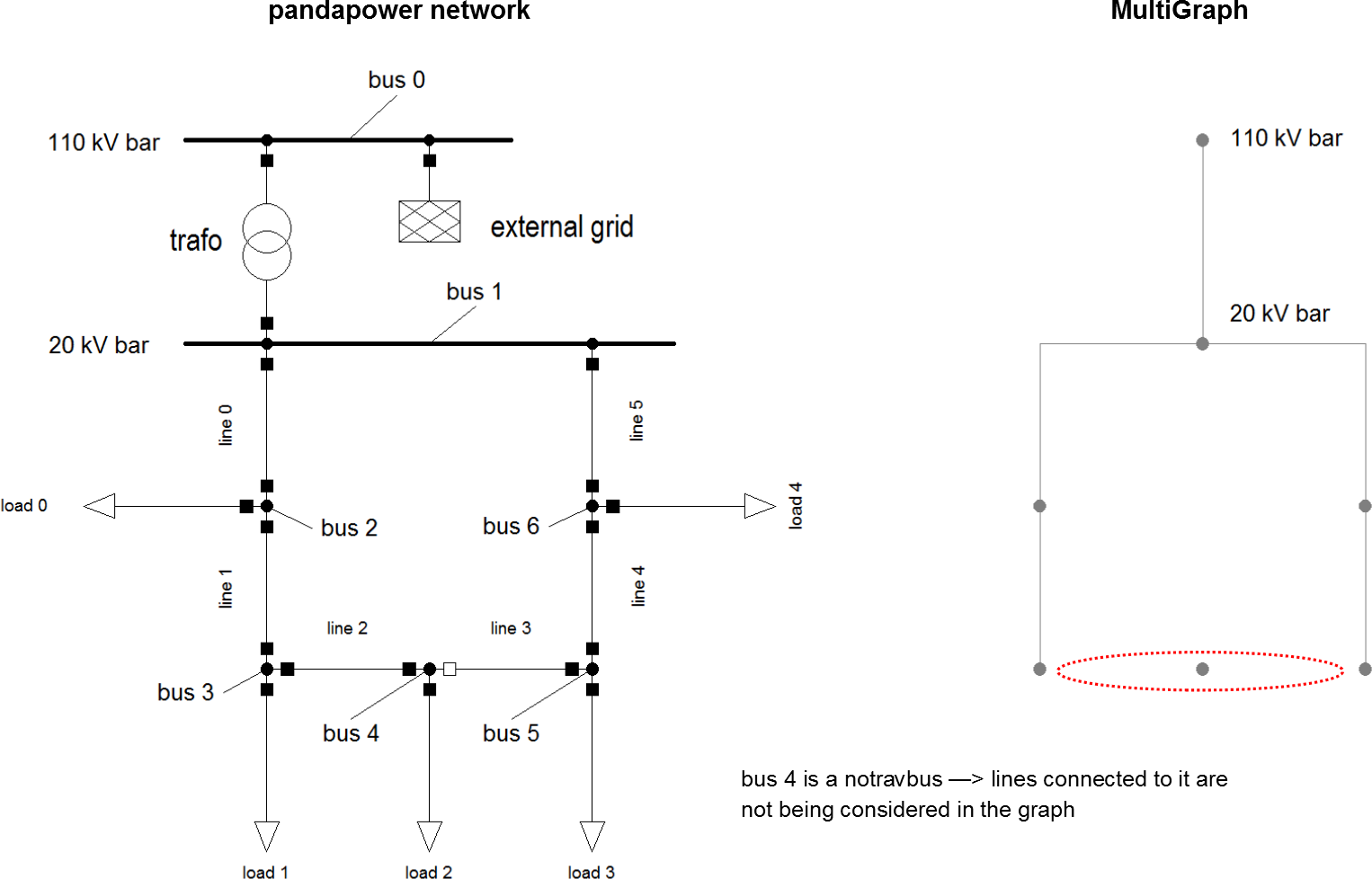Create networkx graph¶

The basis of all topology functions is the conversion of a padapower network into a NetworkX MultiGraph. A MultiGraph is a simplified representation of a network’s topology, reduced to nodes and edges. Busses are being represented by nodes (Note: only buses with in_service = 1 appear in the graph), edges represent physical connections between buses (typically lines or trafos). Multiple parallel edges between nodes are possible.

This is a very simple example of a pandapower network being converted to a MultiGraph. (Note: The MultiGraph’s shape is completely arbitrary since MultiGraphs have no inherent shape unless geodata is provided.)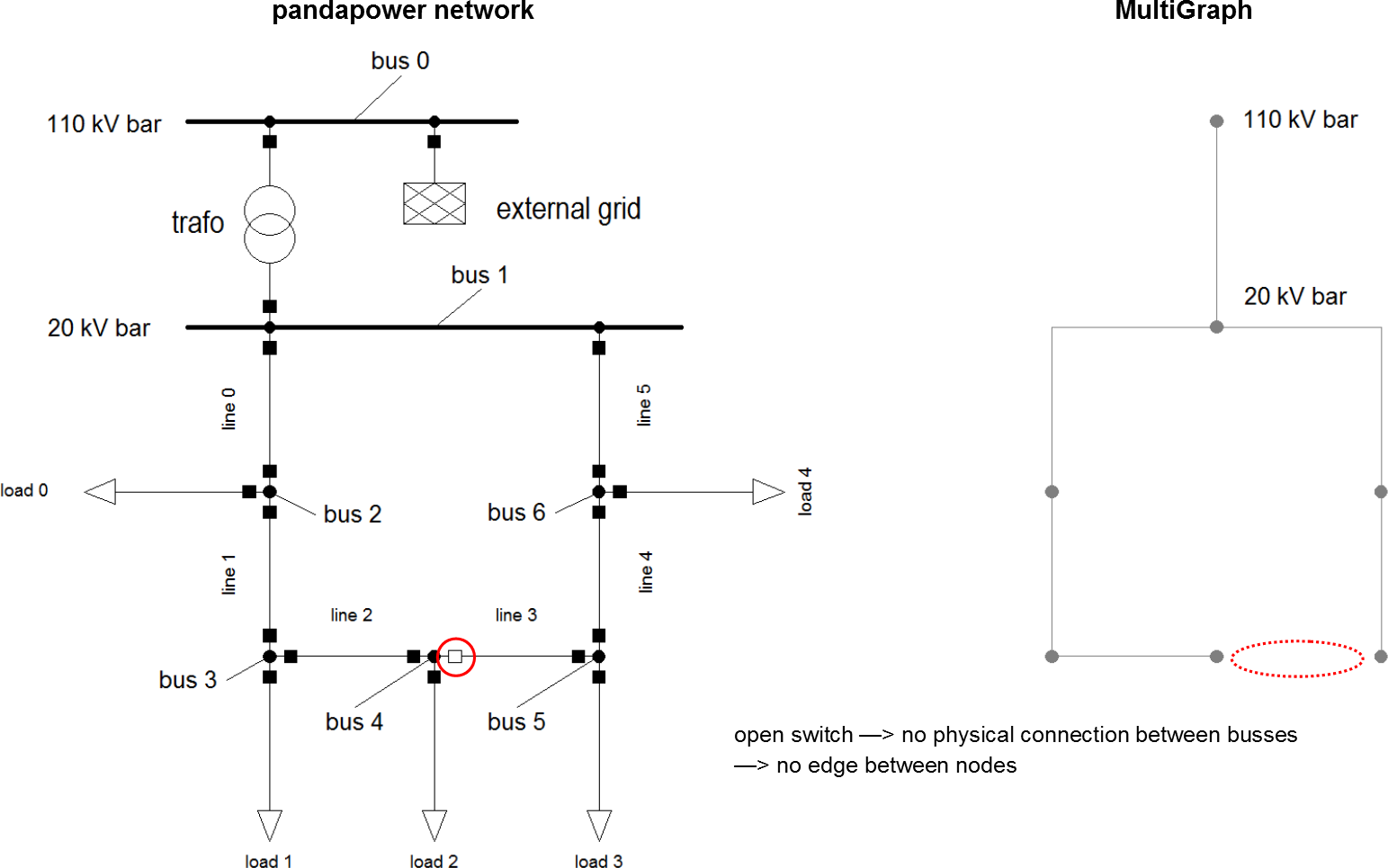Nodes have the same indicees as the buses they originate from. Edges are defined by the nodes they connect. Additionally nodes and edges can hold key/value attribute pairs.

The following attributes get transferred into the MultiGraph:

lines trafos
• from_bus
• to_bus
• length_km
• index
• in_service
• max_i_ka
• hv_bus
• lv_bus
• index
• in_service

Apart from these there are no element attributes contained in the MultiGraph!

Creating a multigraph from a pandapower network

The function create_nxgraph function from the pandapower.topology package allows you to convert a pandapower network into a MultiGraph:

pandapower.topology.create_nxgraph(net, respect_switches=True, include_lines=True, include_trafos=True, include_impedances=True, nogobuses=None, notravbuses=None, multi=True, calc_branch_impedances=False, branch_impedance_unit='ohm')

Converts a pandapower network into a NetworkX graph, which is a is a simplified representation of a network’s topology, reduced to nodes and edges. Busses are being represented by nodes (Note: only buses with in_service = 1 appear in the graph), edges represent physical connections between buses (typically lines or trafos).

INPUT:
net (pandapowerNet) - variable that contains a pandapower network
OPTIONAL:
respect_switches (boolean, True) - True: open switches (line, trafo, bus) are being considered (no edge between nodes)
False: open switches are being ignored

include_lines (boolean, True) - determines, whether lines get converted to edges

include_impedances (boolean, True) - determines, whether per unit impedances
(net.impedance) are converted to edges

include_trafos (boolean, True) - determines, whether trafos get converted to edges

nogobuses (integer/list, None) - nogobuses are not being considered in the graph

notravbuses (integer/list, None) - lines connected to these buses are not being
considered in the graph
multi (boolean, True) - True: The function generates a NetworkX MultiGraph, which allows
multiple parallel edges between nodes False: NetworkX Graph (no multiple parallel edges)

calc_branch_impedances (boolean, False) - detrmines wether impedances are calculated and added as a weight to all branches or not. Impedances can be added in ohm or per unit (see branch_impedance unit parameter)

branch_impedance_unit (str, “ohm”) - defines the unit of the branch impedance for
calc_branch_impedances=True. If it is set to “ohm”, the parameters ‘r_ohm’, ‘x_ohm’ and ‘z_ohm’ are added to each branch. If it is set to “pu”, the parameters are ‘r_pu’, ‘x_pu’ and ‘z_pu’.
OUTPUT:
mg - Returns the required NetworkX graph
EXAMPLE:

import pandapower.topology as top

mg = top.create_nx_graph(net, respect_switches = False) # converts the pandapower network “net” to a MultiGraph. Open switches will be ignored.

Examples

create_nxgraph(net, respect_switches = False)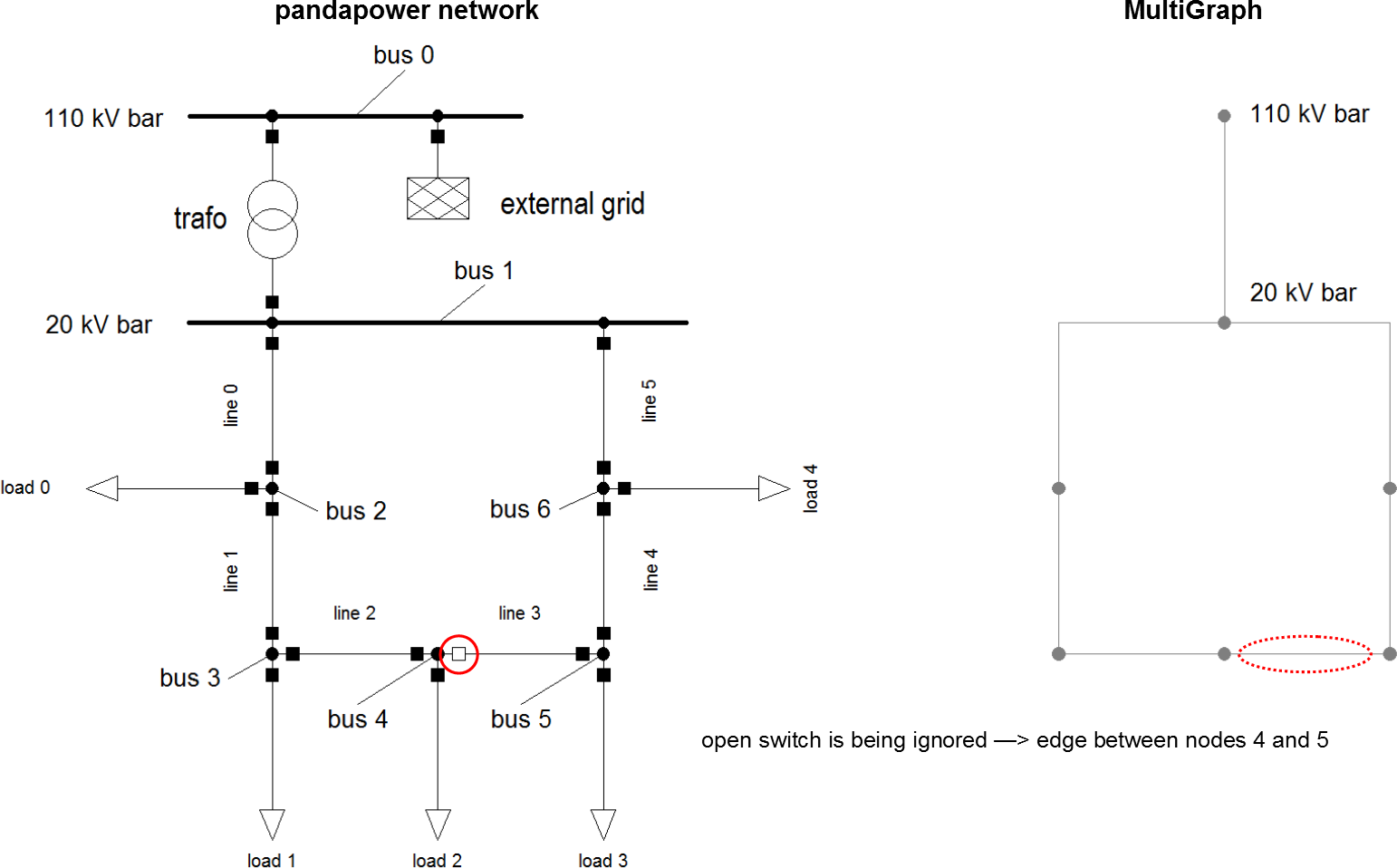create_nxgraph(net, include_lines = False, include_impedances = False)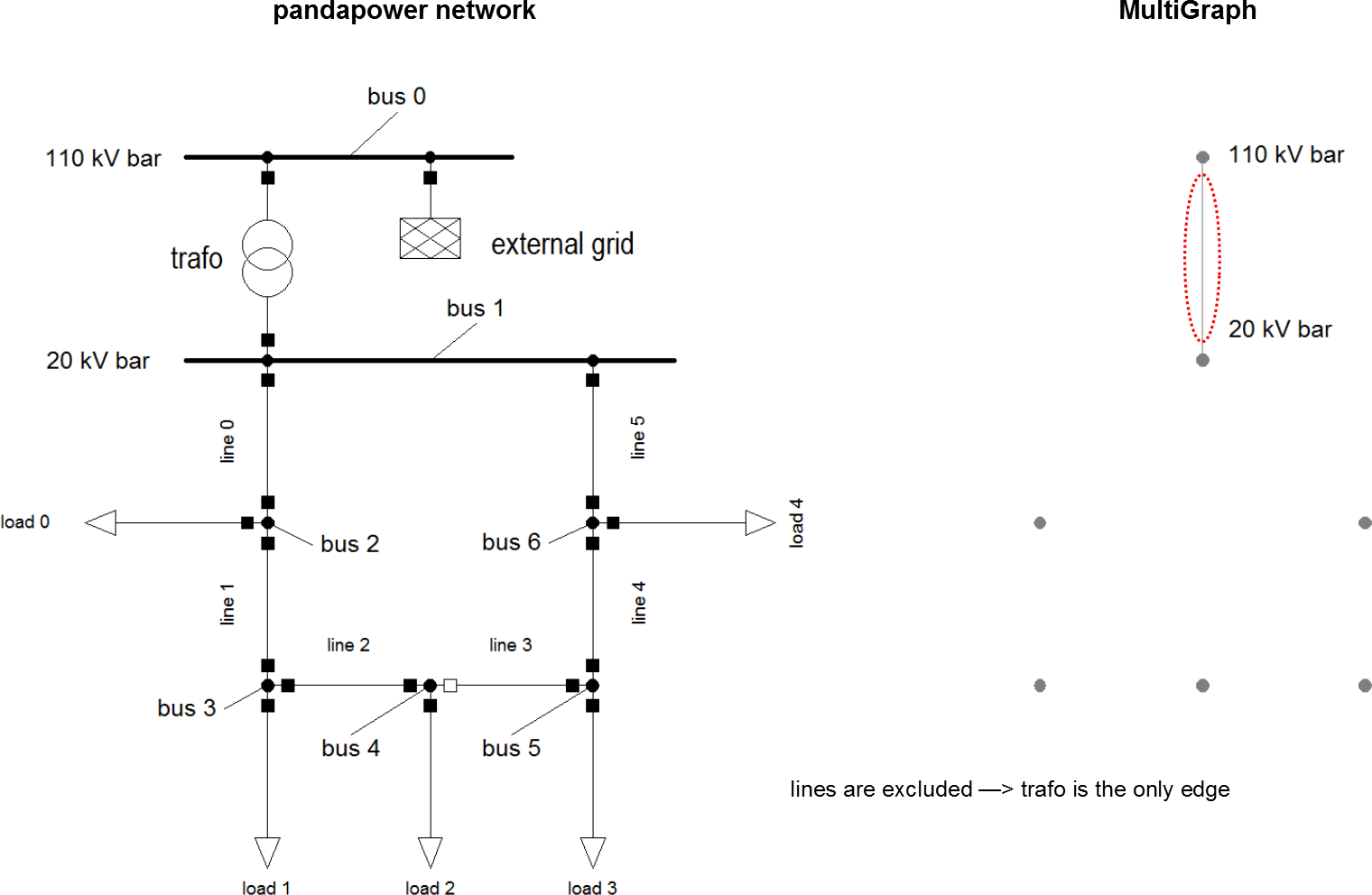create_nxgraph(net, include_trafos = False)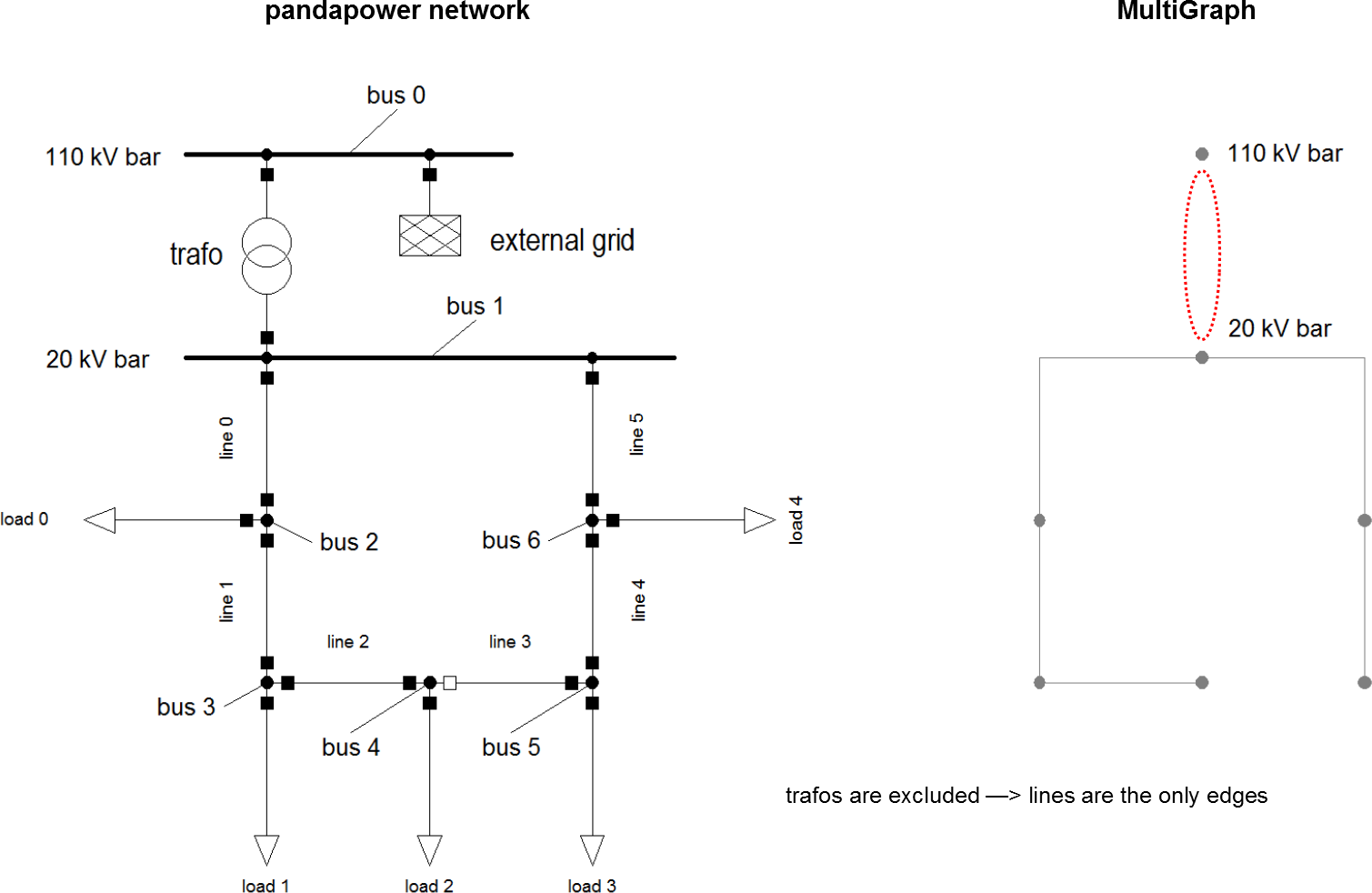create_nxgraph(net, nogobuses = )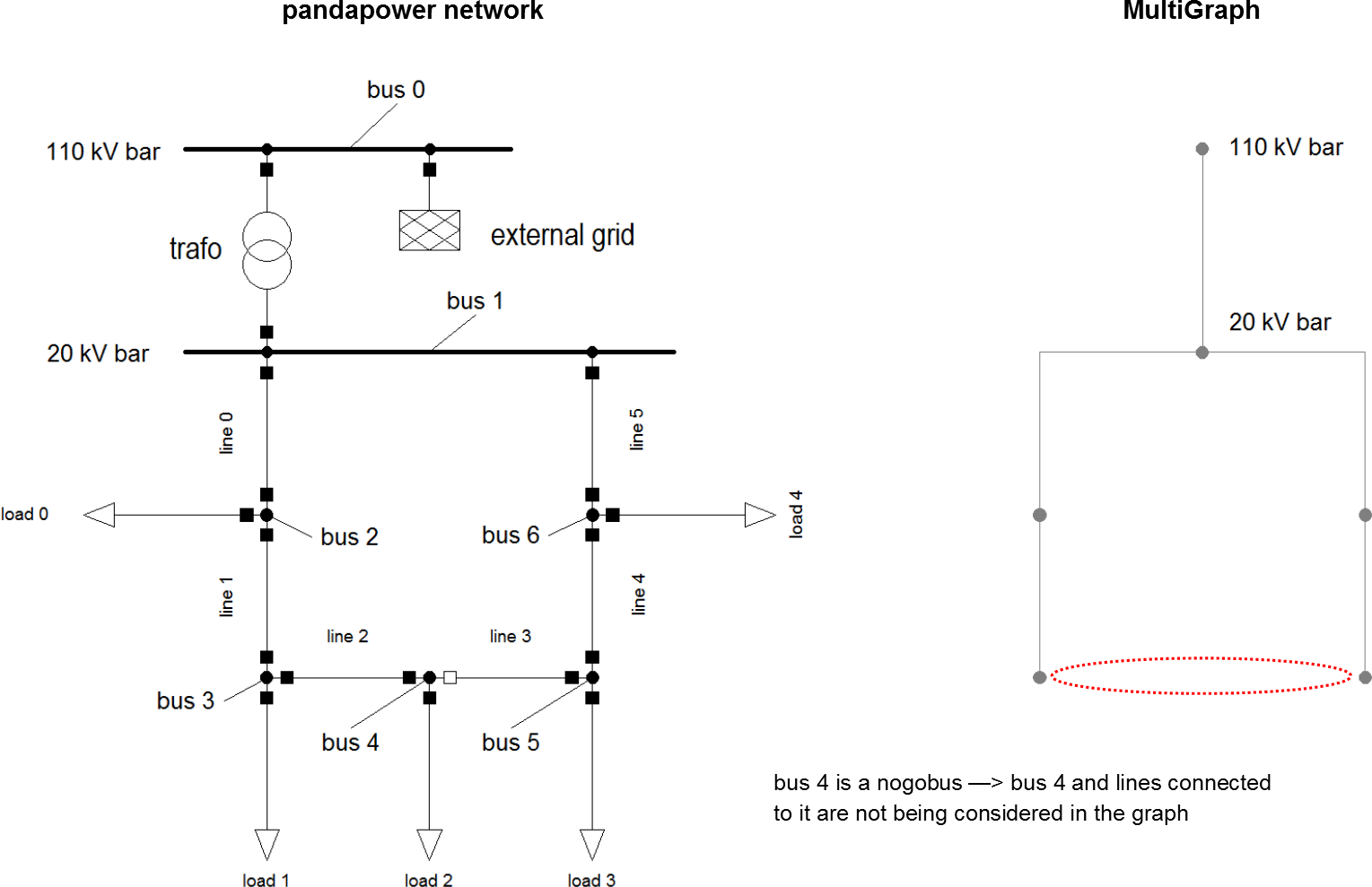create_nxgraph(net, notravbuses = )